Thermos Index

Thermodynamics

Nomenclature..... Polytropic process..... Equation of State..... Relationships between P,V,T..... Adiabatic process.....
Isothermal Process..... Internal Energy..... Constant Volume Process..... Constant Pressure Process.....

Introduction

This page provides a limited notes on thermodynamic relationships useful to mechanical engineers.

Nomenclature

 Identifier Description Units (typical) c p Specific Heat Capacity at Constant pressure kJ/(kg K) c v Specific Heat Capacity at Constant Volume kJ/(kg K) P Absolute Pressure N / m 2 T Absolute Temperature K V volume m 3 m mass kg W Work Output per unit mass kJ/kg M Molecular Weight - R o Universal Gas Constant = 8,31 kJ /(kg mole.K) Q Heat Quantity kJ R Gas Constant = R o / M kJ /kg.K U Internal energy (thermal) kJ γ Ratio cp / cv -

## Thermodynamic Process Relationships

General Polytropic Process

The majority of frictionless processes for ideal gases are called polytropic processes and are in accordance with the following relationship

PV n = constant
That is PV n = c
therefore P = cV -n

Equation of state for and Ideal Gas

PV = mRT

Thermodynamic Relationships between P,V & T

Consider a piston in a frictionless cylinder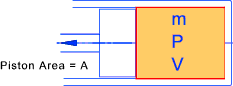The work done on/by the gas in moving the piston δx = (PA)δx = P δ V = δ W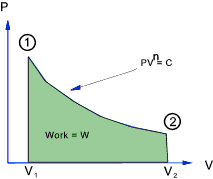The gas is assumed to be expanding in balanced resisted reversible process.
The equation of state for an ideal gas is assumed to apply i.e PV = mRT
The total work done in moving the piston from state 1 to state 2 =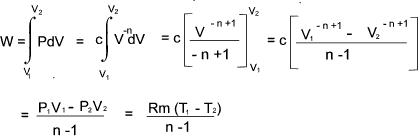For a perfect gas - The relationship between Temperature , Pressure and Volume over a cycle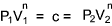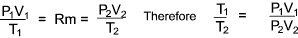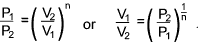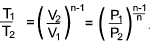For an adiabatic process with no transfer of heat across the system boundary.(Q = 0 )

Consider a fixed mass of gas in a cylinder which is expanding in a reversible manner...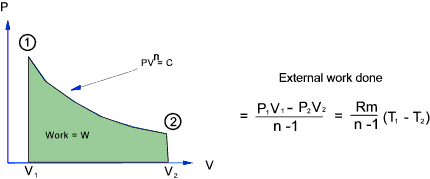For an adiabatic process there is no heat transfer.
Therefore applying the first law of thermodynamics ..heat transfer (= 0) = increase in internal energy +external work done by gas...

Therefore the increase in internal energy = - External work done by gas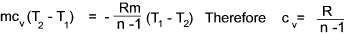It is shown below that cp - cv = R = cv ( cp / cv -1) and therefore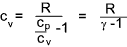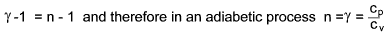γ = 1.4 for Air,  H 2,  O 2, CO, NO, Hcl

γ = 1.3 for CO 2, SO 2,  H 2O, H 2S, N 2O, NH 3, CL 2,  CH 4, C 2H 2, C 2H 4

Isothermal Process

In a isothermal process the temperature = constant and therefore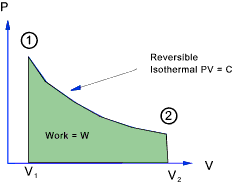PV = c and P = c / V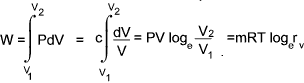Internal Energy, Cp and Cv

Although it is not possible to determine the absolute value of the internal energy of a substance.  The internal energy change between the initial and final equilibrium states of any process is definite and determinable.

It can be easily proved that the internal energy of a fluid depends on the temperature alone and not upon changes in the pressure or volume.

Heating at constant volume....

If a definite mass of gas (m) at constant volume is a closed system is heated from initial conditions P1, V, T1, U1 to P2, V , T2,U2.   As the volume is fixed then no work has been done.    Then in accordance with the First Law of Thermodynamics (δQ = δU + δW ).

mCv (T2 - T1) = (U2 - U1) + 0
or U2 - U2 = mCv (T2 - T1)

Heating at constant pressure....

If a definite mass of gas (m) at constant volume is a closed system is heated from initial conditions P, V1, T1, U1 to P, V1 , T2,U2.   As the volume is fixed then no work has been done.    Then in accordance with the First Law of Thermodynamics (δQ = δU + δW ).

mcp (T2 - T1)
= (U2 - U1) + P (V2 - V1)
= (U2 - U1) + mR (T2 - T1)

mcv (T2 - T1) = U2 - U1 therefore mcp (T2 - T1) = mcv (T2 - T1) + mR (T2 - T1) therefore

cp = cv + R... and ..
cp - cv = R = PV/mT

 Thermodynamic Links Thermodynamics..NASA - Glenn Research center at Series of informative notes on Thermodynamics Thermodynaic properties,property relationships and processes..A very detailed clear study of the subject, (3,3 Mbyte download)

Thermos Index